# Test: Machine Design - 2

## 25 Questions MCQ Test Mechanical Engineering SSC JE (Technical) | Test: Machine Design - 2

Description
Attempt Test: Machine Design - 2 | 25 questions in 50 minutes | Mock test for Mechanical Engineering preparation | Free important questions MCQ to study Mechanical Engineering SSC JE (Technical) for Mechanical Engineering Exam | Download free PDF with solutions
QUESTION: 1

### The design calculations for members subject to fluctuating loads with the same factor of safety yield the most conservative estimates when using

Solution:

Explanation : The soderberg line is a more conservative failure criterion and there is no need to consider even yielding in this case.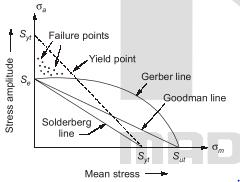QUESTION: 2

### If a shaft made from ductile material is subjected to combined bending and twisting moment, calculations based on which one of the following failure theories will give the most conservative value?

Solution:

Explanation : The concept of equivalent torsional moment is used in the design of shafts on the basis of maximum shear stress theory of failure.  The concept of equivalent bending moment is used in the design of shafts on the basis of maximum principal stress theory of failure

QUESTION: 3

### If the size of a standard specimen for a fatigue testing machine is increased, the endurance limit for the material will

Solution:
QUESTION: 4

Stress concentration in a machine component of ductile materials not so harmful as it is in brittle material because

Solution:
QUESTION: 5

A solid circular shaft is subjected to pure torsion. The ratio of maximum shear stress to maximum normal stress at any point would be

Solution:
QUESTION: 6

Match List-I (Failure theories) with List-II (Figures representing boundaries of these theories) and select the correct answer using the code given below the lists: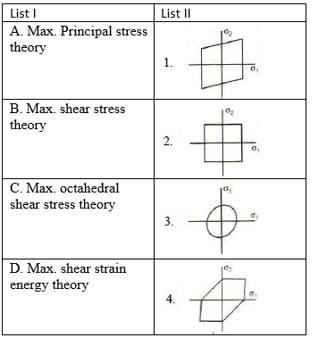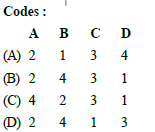Solution:
QUESTION: 7

The maximum distortion energy theory of failure is suitable to predict the failure of which one of the following types of materials ?

Solution:
QUESTION: 8

Which one of the following figures represents the maximum shear stress theory of TRESCA criterion ?

Solution:
QUESTION: 9

Match List-I (Theory of Failure) with List-II (Predicted Ratio of Shear Stress to Direct Stress at yield Condition for Steel Specimen) select the correct answer using the code given below the lists: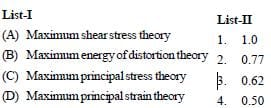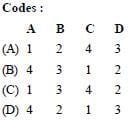Solution:
QUESTION: 10

Disruptive strength is the maximum strength of a metal, when

Solution:
QUESTION: 11

Who postulated the maximum distortion energy theory?

Solution:
QUESTION: 12

A rotating shaft carrying a unidirectional transverse load is subjected to :

Solution:
QUESTION: 13

In the design of connecting rod small end bearing, the value of permissible bearing pressure to be used is :

Solution:
QUESTION: 14

For bolts of uniform strength, the shank diameter is made equal to

Solution:
QUESTION: 15

A butt welded joint, subjected to tensile force P is shown in the given figure, l = length of the weld (in mm) h = throat of the butt weld (in mm) and H is the total height of weld including reinforcement. The average tensile stress st in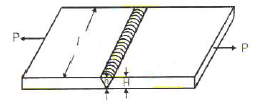Solution:
QUESTION: 16

In the welded joint shown in the given figure if the weld at B has thicker fillets than at A then load carrying capacity P0 of the joint will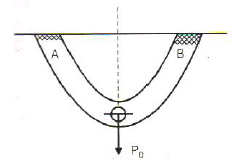Solution:
QUESTION: 17

When a nut is tightened by placing a washer below it the bolt will be subjected to

Solution:
QUESTION: 18

The rivet head used for boiler plate riveting is usually

Solution:
QUESTION: 19

The piston rod and the crosshead in a steam engine are usually connected by means of

Solution:
QUESTION: 20

For longitudinal joint in boiler, the type of joint used is

Solution:
QUESTION: 21

For the bracket bolted as shown in the above figure, the bolts will develop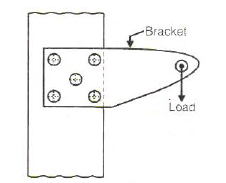Solution:
QUESTION: 22

Match List-I (Applications) with List-II (Joints) and select the correct answer using the code given below the lists: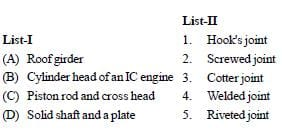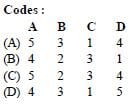Solution:
QUESTION: 23

Match List-I (Type of joints) List-II (Mode of connecting member) and select the correct answer using the code given below the lists: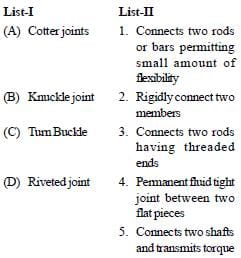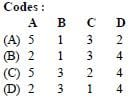Solution:
QUESTION: 24

Match List-I with List-II and select the correct answer using the code given below the lists: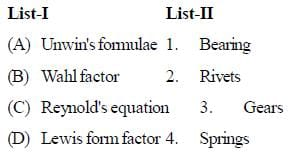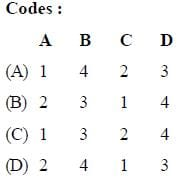Solution:
QUESTION: 25

Which of the following property is affected by heat treatment?

Solution:

Heat treatment involves changes in the micro structure and hence all the internal properties are effected.Use Code STAYHOME200 and get INR 200 additional OFF Use Coupon Code